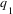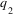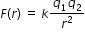# MA.912.C.1.3

Find limits of rational functions that are undefined at a point.

### Examples

The magnitude of the force between two positive charges,and, can be described by the following function:, where k is Coulomb’s constant and r is the distance between the two charges. Find the limit as r approaches 0 of the function F(r). interpret the answer in terms of the context.
General Information
Subject Area: Mathematics (B.E.S.T.)
Strand: Calculus
Status: State Board Approved

## Related Courses

This benchmark is part of these courses.
1202300: Calculus Honors (Specifically in versions: 2014 - 2015, 2015 - 2022, 2022 and beyond (current))

## Related Access Points

Alternate version of this benchmark for students with significant cognitive disabilities.

## Related Resources

Vetted resources educators can use to teach the concepts and skills in this benchmark.

## Tutorial

Redefining a Function by Finding a Limit to Make the Function Continuous:

In this video students are introduced to an algebraic technique for rewriting a rational function in order to find a limit of the function.

Type: Tutorial

## Student Resources

Vetted resources students can use to learn the concepts and skills in this benchmark.

## Tutorial

Redefining a Function by Finding a Limit to Make the Function Continuous:

In this video students are introduced to an algebraic technique for rewriting a rational function in order to find a limit of the function.

Type: Tutorial

## Parent Resources

Vetted resources caregivers can use to help students learn the concepts and skills in this benchmark.